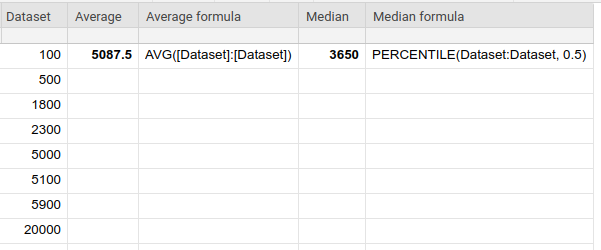A personal blog.

# The Median in Smartsheet

If you needed the median function in Smartsheet, but couldn't find it, don't get upset. That function, indeed, is not present among supported functions; however, there is the percentile function available in Smartsheet. And the good news is that the median is just another name for the 50th percentile.

To calculate the median in Smartsheet, use this formula:

=PERCENTILE(data, 0.5)

Now as we touched on the aggregation topic, let me also talk briefly about the difference between an average and the median - just in case you didn't know.

Average, or the arithmetic mean, is a sum of all values divided by the number of values. Because it takes every value into account, it tends to be skewed by extremes. Sometimes this is exactly what you need. If your intention, however, is to express what's typical, the median does a better job because it's not sensitive to outliers in a data set.

Here is an example to show how the average differs from the median for a particular dataset: